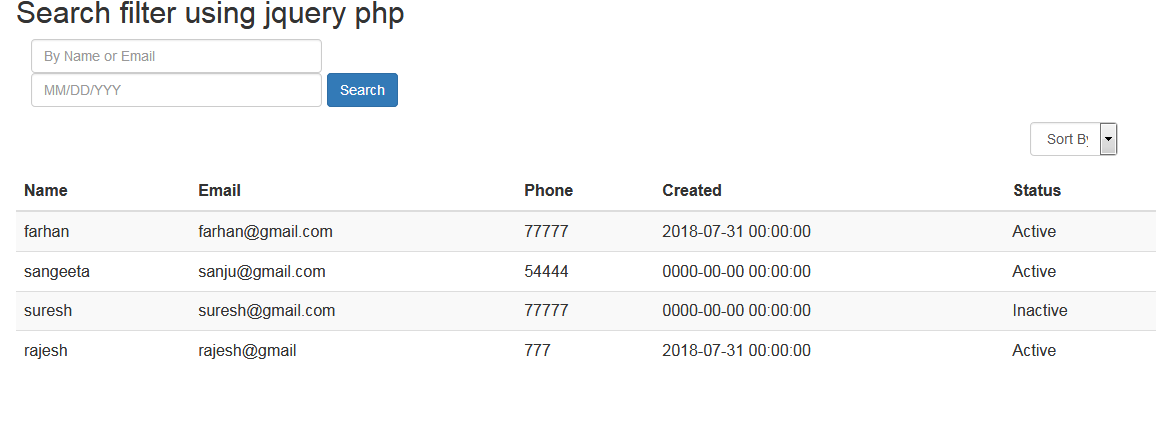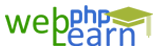Apply for Zend Framework Certification Training

# Jquery```step - 1
<script src="http://maxcdn.bootstrapcdn.com/bootstrap/3.3.6/js/bootstrap.min.js"></script>
<script type="text/javascript" src="https://cdnjs.cloudflare.com/ajax/libs/bootstrap-datepicker/1.4.1/js/bootstrap-datepicker.min.js"></script>

<style>
.form-control{float: left;width: 70%;}
.btn{float: left;margin-left: 5px;}
.container{position: relative;}
position: absolute;
left: 0;
top: 0;
right: 0;
bottom: 0;
z-index: 2;
background: rgba(255,255,255,0.7);
}
.overlay-content {
position: absolute;
transform: translateY(-50%);
-webkit-transform: translateY(-50%);
-ms-transform: translateY(-50%);
top: 50%;
left: 0;
right: 0;
text-align: center;
color: #555;
}
</style>
<div class="container">
<h2>Search filter using jquery php</h2>
<div class="bootstrap-iso">
<div class="container-fluid">
<form method="post">
<div class="form-group pull-left">
<input type="text" class="search form-control" id="searchInput" placeholder="By Name or Email">
<input class="form-control" id="date" name="date" placeholder="MM/DD/YYY" type="text"/>
<input type="button" class="btn btn-primary" value="Search" onclick="getUsers('search',\$('#searchInput').val())"/>
</div>
</form>
</div>
</div>
<div class="form-group pull-right">
<select class="form-control" onchange="getUsers('sort',this.value)">
<option value="">Sort By</option>
<option value="asc">Ascending</option>
<option value="desc">Descending</option>
<option value="active">Active</option>
<option value="inactive">Inactive</option>
</select>
</div>
<table class="table table-striped">
<tr>
<th>Name</th>
<th>Email</th>
<th>Phone</th>
<th>Created</th>
<th>Status</th>
</tr>
<tbody id="userData">
<?php
include 'DB.php';
\$db = new DB();
\$users = \$db->getRows('users_new',array('order_by'=>'id DESC'));
if(!empty(\$users)){ \$count = 0;
foreach(\$users as \$user){ \$count++;
?>
<tr>
<td><?php echo \$user['name']; ?></td>
<td><?php echo \$user['email']; ?></td>
<td><?php echo \$user['phone']; ?></td>
<td><?php echo \$user['created']; ?></td>
<td><?php echo (\$user['status'] == 1)?'Active':'Inactive'; ?></td>
</tr>
<?php } }else{ ?>
<tr><td colspan="5">No user(s) found...</td></tr>
<?php } ?>
</tbody>
</table>
</div>
<script>
function getUsers(type,val){
var start_date = \$("#date").val();
\$.ajax({
type: 'POST',
url: 'getData.php',
data: 'type='+type+'&val='+val+'&start_date='+start_date,
beforeSend:function(html){
},
success:function(html){
\$('#userData').html(html);
}
});
}
</script>
<script>
var date_input=\$('input[name="date"]'); //our date input has the name "date"
var container=\$('.bootstrap-iso form').length>0 ? \$('.bootstrap-iso form').parent() : "body";
var options={
format: 'yyyy-mm-dd',
container: container,
todayHighlight: true,
autoclose: true,
};
date_input.datepicker(options);
})
</script>

Step -2
<?php
include 'DB.php';
\$db = new DB();
\$tblName = 'users_new';
\$conditions = array();
if(!empty(\$_POST['type']) && !empty(\$_POST['val'])){
if(\$_POST['type'] == 'search'){
\$conditions['search'] = array('name'=>\$_POST['val'],'email'=>\$_POST['val'],'created'=>\$_POST['start_date']);
\$conditions['order_by'] = 'id DESC';
}elseif(\$_POST['type'] == 'sort'){
\$sortVal = \$_POST['val'];
\$sortArr = array(
'new' => array(
'order_by' => 'created DESC'
),
'asc'=>array(
'order_by'=>'name ASC'
),
'desc'=>array(
'order_by'=>'name DESC'
),
'active'=>array(
'where'=>array('status'=>'1')
),
'inactive'=>array(
'where'=>array('status'=>'0')
)
);
\$sortKey = key(\$sortArr[\$sortVal]);
\$conditions[\$sortKey] = \$sortArr[\$sortVal][\$sortKey];
}
}else{
\$conditions['order_by'] = 'id DESC';
}
\$users = \$db->getRows(\$tblName,\$conditions);
if(!empty(\$users)){
\$count = 0;
foreach(\$users as \$user): \$count++;
echo '<tr>';
echo '<td>'.\$user['name'].'</td>';
echo '<td>'.\$user['email'].'</td>';
echo '<td>'.\$user['phone'].'</td>';
echo '<td>'.\$user['created'].'</td>';
\$status = (\$user['status'] == 1)?'Active':'Inactive';
echo '<td>'.\$status.'</td>';
echo '</tr>';
endforeach;
}else{
echo '<tr><td colspan="5">No user(s) found...</td></tr>';
}
exit;
step - 3
<?php
/*
* DB Class
* This class is used for database related (connect and fetch) operations
* @author    CodexWorld.com
* @url       http://www.codexworld.com
*/
class DB{
private \$dbHost     = "localhost";
private \$dbName     = "ajax";

public function __construct(){
if(!isset(\$this->db)){
// Connect to the database
if(\$conn->connect_error){
die("Failed to connect with MySQL: " . \$conn->connect_error);
}else{
\$this->db = \$conn;
}
}
}

/*
* Returns rows from the database based on the conditions
* @param string name of the table
* @param array select, where, search, order_by, limit and return_type conditions
*/
public function getRows(\$table,\$conditions = array()){
\$sql = 'SELECT ';
\$sql .= array_key_exists("select",\$conditions)?\$conditions['select']:'*';
\$sql .= ' FROM '.\$table;
//echo \$sql;
if(array_key_exists("where",\$conditions)){
\$sql .= ' WHERE ';
\$i = 0;
foreach(\$conditions['where'] as \$key => \$value){
\$pre = (\$i > 0)?' AND ':'';
\$sql .= \$pre.\$key." = '".\$value."'";
\$i++;
}
}

if(array_key_exists("search",\$conditions)){
\$sql .= (strpos(\$sql, 'WHERE') !== false)?'':' WHERE ';
\$i = 0;
foreach(\$conditions['search'] as \$key => \$value){
\$pre = (\$i > 0)?' AND ':'';
\$sql .= \$pre.\$key." LIKE '%".\$value."%'";
\$i++;
}
}
//echo \$sql;
if(array_key_exists("order_by",\$conditions)){
\$sql .= ' ORDER BY '.\$conditions['order_by'];
}

if(array_key_exists("start",\$conditions) && array_key_exists("limit",\$conditions)){
\$sql .= ' LIMIT '.\$conditions['start'].','.\$conditions['limit'];
}elseif(!array_key_exists("start",\$conditions) && array_key_exists("limit",\$conditions)){
\$sql .= ' LIMIT '.\$conditions['limit'];
}
//echo \$sql;
\$result = \$this->db->query(\$sql);

if(array_key_exists("return_type",\$conditions) && \$conditions['return_type'] != 'all'){
switch(\$conditions['return_type']){
case 'count':
\$data = \$result->num_rows;
break;
case 'single':
\$data = \$result->fetch_assoc();
break;
default:
\$data = '';
}
}else{
if(\$result->num_rows > 0){
while(\$row = \$result->fetch_assoc()){
\$data[] = \$row;
}
}
}
return !empty(\$data)?\$data:false;
}
}                                    ```

`{{questionlistdata.blog_question_description}}`
`{{answer.blog_answer_description  }}`